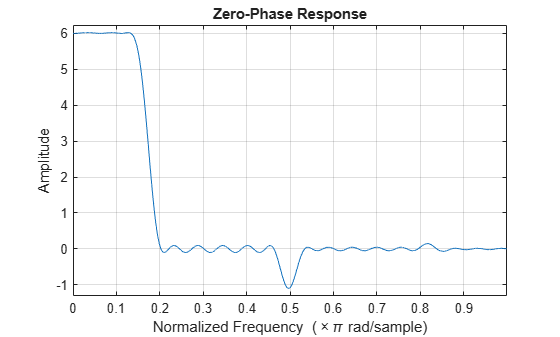# fireqint

Equiripple FIR interpolators

## Syntax

```b = fireqint(n,l,alpha) b = fireqint(n,l,alpha,w) b = fireqint('minorder',l,alpha,r) b = fireqint({'minorder',initord},l,alpha,r) ```

## Description

`b = fireqint(n,l,alpha)` designs an FIR equiripple filter useful for interpolating input signals. `n` is the filter order and it must be an integer. `l`, also an integer, is the interpolation factor. `alpha` is the bandlimitedness factor, identical to the same feature in `intfilt`.

`alpha` is inversely proportional to the transition bandwidth of the filter. It also affects the bandwidth of the don't-care regions in the stopband. Specifying `alpha` allows you to control how much of the Nyquist interval your input signal occupies. This can be beneficial for signals to be interpolated because it allows you to increase the transition bandwidth without affecting the interpolation, resulting in better stopband attenuation for a given `l`. If you set `alpha` to 1, `fireqint` assumes that your signal occupies the entire Nyquist interval. Setting `alpha` to a value less than one allows for don't-care regions in the stopband. For example, if your input occupies half the Nyquist interval, you could set `alpha` to 0.5.

The signal to be interpolated is assumed to have zero (or negligible) power in the frequency band between (`alpha`*π) and π. `alpha` must therefore be a positive scalar between 0 and 1. `fireqint` treat such bands as don't-care regions for assessing filter design.

`b = fireqint(n,l,alpha,w)` allows you to specify a vector of weights in `w`. The number of weights required in `w` is given by 1 + `floor`(`l`/2). The weights in `w` are applied to the passband ripple and stopband attenuations. Using weights (values between 0 and 1) enables you to specify different attenuations in different parts of the stopband, as well as providing the ability to adjust the compromise between passband ripple and stopband attenuation.

`b = fireqint('minorder',l,alpha,r)` allows you to design a minimum-order filter that meets the design specifications. `r` is a vector of maximum deviations or ripples from the ideal filter magnitude response. When you use the input argument `minorder`, you must provide the vector `r`. The number of elements required in `r` is given by 1 + `floor`(`l/`2).

`b = fireqint({'minorder',initord},l,alpha,r)` adds the argument `initord` so you can provide an initial estimate of the filter order. Any positive integer is valid here. Again, you must provide `r`, the vector of maximum deviations or ripples, from the ideal filter magnitude response.

## Examples

collapse all

Design a minimum order interpolation filter with `l` set to 6, and `alpha` set to 0.8. A vector of ripples must be supplied with the input argument `minorder`.

`b = fireqint('minorder',6,.8,[0.01 .1 .05 .02]);`

Create a polyphase interpolation filter.

`hm = dsp.FIRInterpolator(6,'Numerator',b)`
```hm = dsp.FIRInterpolator with properties: NumeratorSource: 'Property' Numerator: [1x70 double] InterpolationFactor: 6 Show all properties ```

Here is the zerophase response of the interpolator.

`zerophase(hm);`